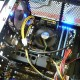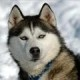# [OpenCV] detectMultiScale: output detection score

OpenCV provides quite decent implementation of the Viola-Jones Face detector.

A quick example looks like this (OpenCV 2.4.5 tested):

```// File: main.cc
#include

using namespace cv;

int main(int argc, char **argv) {

const float scale_factor(1.2f);
const int min_neighbors(3);

for (int i = 1; i < argc; i++) {

equalizeHist(img, img);
vector objs;

for (int n = 0; n < objs.size(); n++) {
rectangle(img_color, objs[n], Scalar(255,0,0), 8);
}
imshow("VJ Face Detector", img_color);
waitKey(0);
}
}

return 0;
}
```
```g++ -std=c++0x -I/usr/local/include `pkg-config --libs opencv` main.cc -o main
```

The detection results are as shown below:For more serious user, it would be nice to have a detection result for each detected face.
The OpenCV provides a overloaded function designed for this usage which is lack of detailed documentation:

```vector reject_levels;
vector level_weights;
cascade.detectMultiScale(img, objs, reject_levels, level_weights, scale_factor, min_neighbors);
```

The reject_levels and level_weights will keep being empty until you write it like this (The whole file):

```// File: main.cc
#include

using namespace cv;

int main(int argc, char **argv) {

const float scale_factor(1.2f);
const int min_neighbors(3);

for (int i = 1; i < argc; i++) {

equalizeHist(img, img);
vector objs;
vector reject_levels;
vector level_weights;
cascade.detectMultiScale(img, objs, reject_levels, level_weights, scale_factor, min_neighbors, 0, Size(), Size(), true);

for (int n = 0; n < objs.size(); n++) {
rectangle(img_color, objs[n], Scalar(255,0,0), 8);
putText(img_color, std::to_string(level_weights[n]),
Point(objs[n].x, objs[n].y), 1, 1, Scalar(0,0,255));
}
imshow("VJ Face Detector", img_color);
waitKey(0);
}
}

return 0;
}
```

However, this will give you a large number of detected rectangles:This is because OpenCV skips the step of filtering out the overlapped small rectangles. I have no idea whether this is by design. But output likes this would not be helpful at least in my own case.

So we would need to make our own changes in the OpenCV's source code.
There are different ways to design detection score, such as
"In the OpenCV implementation, stage_sum is computed and compared against the i stage_threshold for each stage to accept/reject a candidate window. We define the detection score for a candidate window as K*stage_when_rejected + stage_sum_for_stage_when_rejected. If a window is accepted by the cascade, we just K*last_stage + stage_sum_for_last_stage. Choosing K as a large value e.g., 1000, we ensure that windows rejected at stage i have higher score than those rejected at stage i-1." from http://vis-www.cs.umass.edu/fddb/faq.html

Actually, I found a straightforward design of detection score works well in my own work. In the last stage of the face detector in OpenCV, detection rectangles are grouped into clustered to eliminated small overlapped rectangles while keeping the most potential rectangles. The number of final detected faces is at most same as the number of clusters. So we can simply use the number of rectangles grouped into the cluster as the detection score of the associated final rectangle, which may not be accurate but could work.

To make this change, in OpenCV-2.4.5, find the file modules/objdetect/src/cascadedetect.cpp (line 200)

```// modules/objdetect/src/cascadedetect.cpp (line 200)
// int n1 = levelWeights ? rejectLevels[i] : rweights[i]; //< comment out this line
int n1 = rweights[i]; //< the change
```

We then modify the file main.cc accordingly:

```// File: main.cc
#include

using namespace cv;

int main(int argc, char **argv) {

const float scale_factor(1.2f);
const int min_neighbors(3);

for (int i = 1; i < argc; i++) {

equalizeHist(img, img);
vector objs;
vector reject_levels;
vector level_weights;
cascade.detectMultiScale(img, objs, reject_levels, level_weights, scale_factor, min_neighbors, 0, Size(), Size(), true);

for (int n = 0; n < objs.size(); n++) {
rectangle(img_color, objs[n], Scalar(255,0,0), 8);
putText(img_color, std::to_string(reject_levels[n]),
Point(objs[n].x, objs[n].y), 1, 1, Scalar(0,0,255));
}
imshow("VJ Face Detector", img_color);
waitKey(0);
}
}

return 0;
}
```

And we can have the detection scores like this:1.2.••3.••••4.•5.••••6.